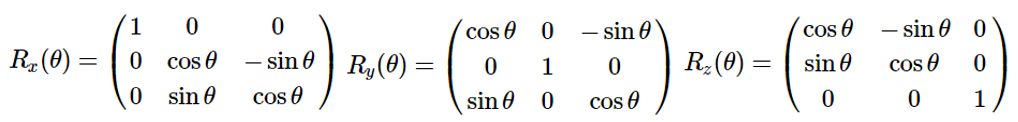## Introduction to Symmetry

Aim: provide a systematic treatment of symmetry in chemical systems within the mathematical framework known as group theory. Once we have classified the symmetry of a molecule, group theory provides a powerful set of tools that provide us with considerable insight into many of its chemical and physical properties.

Some applications of group theory that will be covered in this course include:

• Predicting whether a given molecule will be chiral ([ˈkaɪrəl] 手性的), or polar.
• Examining chemical bonding and visualising molecular orbitals.
• Predicting whether a molecule may absorb light of a given polarisation, and which spectroscopic transitions may be excited if it does.
• Investigating the vibrational motions of the molecule.

## Symmetry Operations and Symmetry Elements

Symmetry Operation:
(1) An action that leaves an object looking the same after it has been carried out.
(2) Each symmetry operation has a corresponding symmetry element, which is the axis, plane, line or point with respect to which the symmetry operation is carried out.

Example: H2O，是轴对称(Axial symmetry)，沿着对称轴(a symmetry axis)旋转180度和原来的状态重合，另外有两个对称面(a plane of symmetry)，而且都之前的对称轴都在对称面上。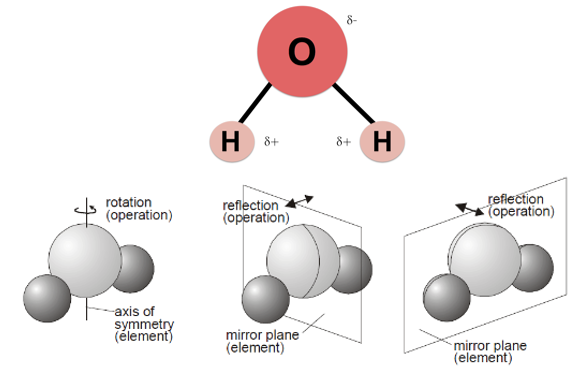Symmetry Elements: ( a molecule may possess)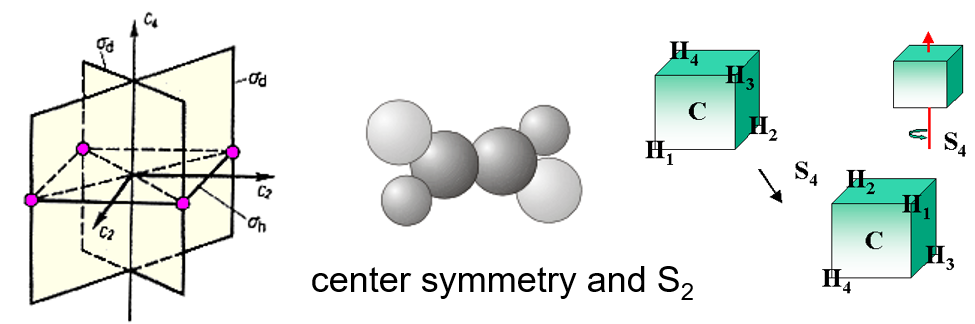• $$E$$ - the identity. The identity operation consists of doing nothing, and the corresponding symmetry element is the entire molecule. Every molecule has at least this element.
• $$C_n$$ - an $$n$$-fold axis of rotation. 水分子有$$C_2$$ axis. 一些molecules有more than one $$C_n$$轴，其中with the highest value of $$n$$ is called the principal axis. Note that by convention rotations are counterclockwise about the axis.
• $$\sigma$$ -  a plane of symmetry. In a molecule that also has an axis of symmetry, a mirror plane that includes the axis is called a vertical mirror plane and is labeled $$\sigma_{v}$$ , while one perpendicular to the axis is called a horizontal mirror plane and is labeled $$\sigma_{h}$$. A vertical mirror plane that bisects (二等分) the angle between two $$C_2$$ axes is called a dihedral (有两个平面的) mirror plane, $$\sigma_{d}$$.
• $$i$$ - a center of symmetry. Inversion through the center of symmetry leaves the molecule unchanged. Inversion consists of passing each point through the center of inversion and out to the same distance on the other side of the molecule.
• $$S_n$$ - an n-fold improper rotation axis (also called a rotary-reflection axis). The rotary reflection operation consists of rotating through an angle 360°/$$n$$ about the axis, followed by reflecting in a plane perpendicular to the axis. Note that $$S_1$$ is the same as reflection and $$S_2$$ is the same as inversion. The molecule shown above has two $$S_2$$ axes.
$$S_n$$ = rotation of 360°/$$n$$ + perpendicular mirror reflection

Proper Symmetry Operations versus Improper Symmetry Operations
The identity $$E$$ and rotations $$C_n$$ are symmetry operations that could actually be carried out on a molecule. For this reason they are called proper symmetry operations. Reflections, inversions and improper rotations can only be imagined (it is not actually possible to turn a molecule into its mirror image or to invert it without some fairly drastic rearrangement of chemical bonds) and as such, are termed improper symmetry operations.

## Symmetry Classification of Molecules- Point Groups

It is only possible for certain combinations of symmetry elements to be present in a molecule (or any other object). As a result, we may group together molecules that possess the same symmetry elements and classify molecules according to their symmetry. These groups of symmetry elements are called point groups (due to the fact that there is at least one point in space that remains unchanged no matter which symmetry operation from the group is applied). There are two systems of notation for labeling symmetry groups, called the Schoenflies and Hermann-Mauguin (or International) systems. The symmetry of individual molecules is usually described using the Schoenflies notation, and we shall be using this notation for the remainder of the course.

Some of the point groups share their names with symmetry operations, so be careful you do not mix up the two. It is usually clear from the context which one is being referred to.

Molecular Point Groups

(1) $$C_1$$ - contains only the identity. 比如CHFClBr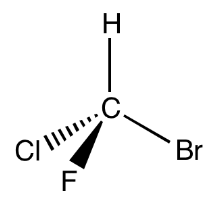(2) $$C_i$$ - contains the identity $$E$$ and a center of inversion $$i$$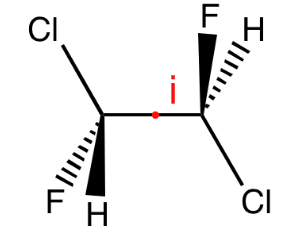(3) $$C_S$$ - contains the identity $$E$$ and a plane of reflection $$\sigma$$(4) $$C_n$$ - contains the identity and an $$n$$-fold axis of rotation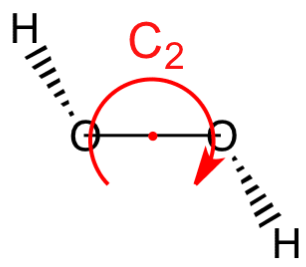(5) $$C_{nv}$$ - contains the identity, an $$n$$-fold axis of rotation, and $$n$$ vertical mirror planes $$\sigma_v$$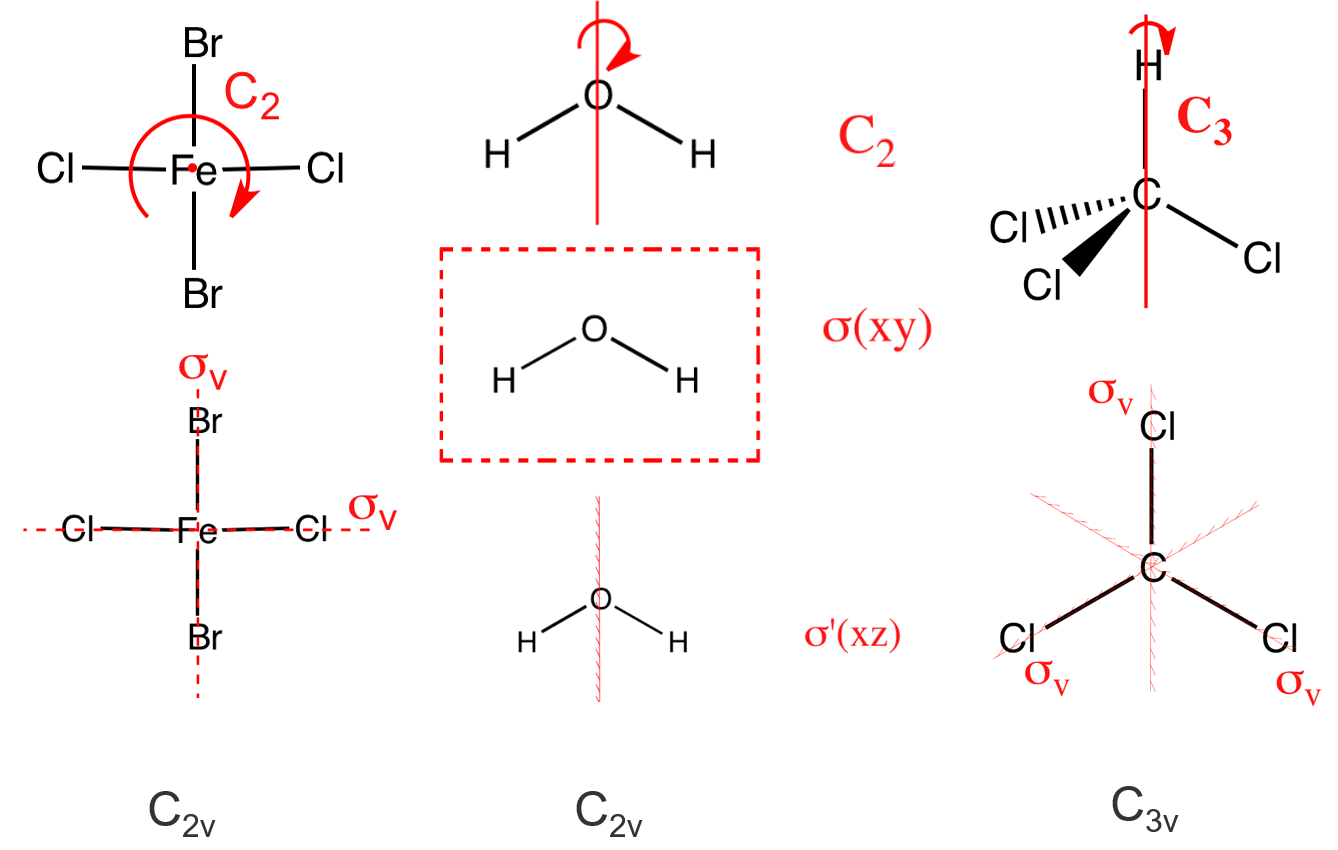(6) $$C_{nh}$$ - contains the identity, an $$n$$-fold axis of rotation, and a horizontal reflection plane $$\sigma_{h}$$ (note that in $$C_{2 h}$$ this combination of symmetry elements automatically implies a center of inversion)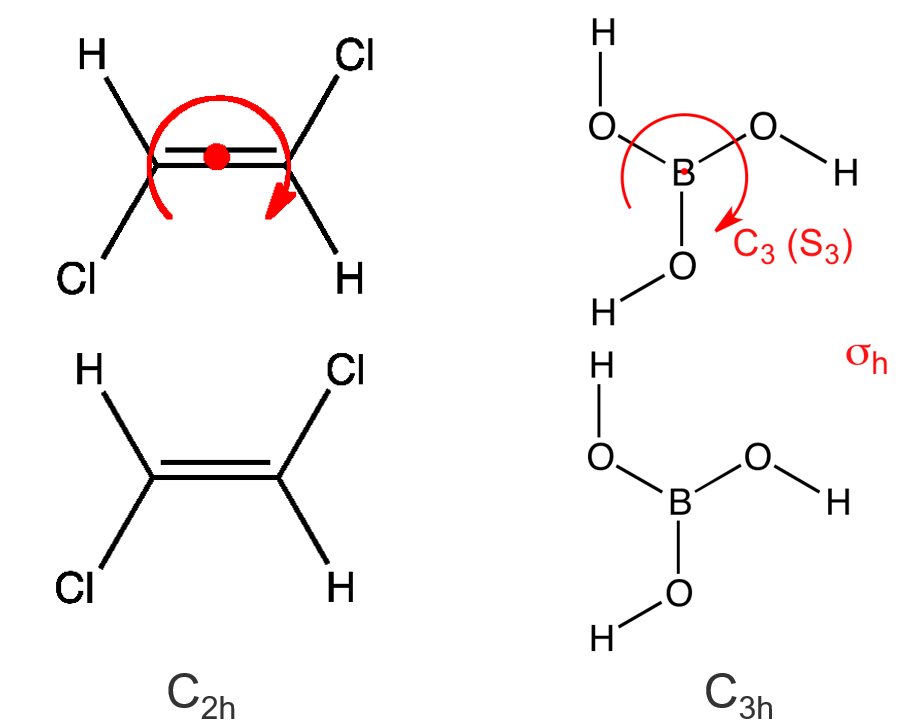(7) $$D_n$$- contains the identity, an $$n$$-fold axis of rotation, and $$n$$ 2-fold rotations about axes perpendicular to the principal axis ($$C_n$$). 下图是$$D_3$$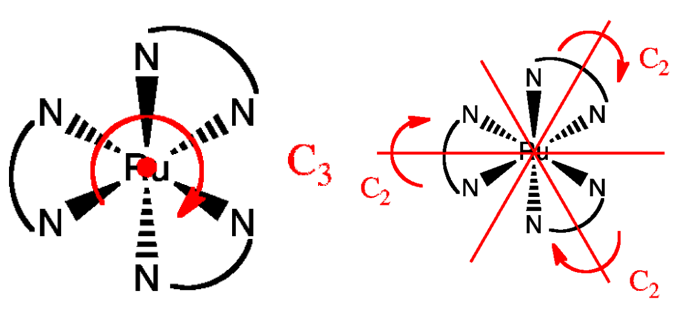(8) $$D_{nh}$$ - contains the same symmetry elements as $$D_n$$ with the addition of a horizontal mirror plane.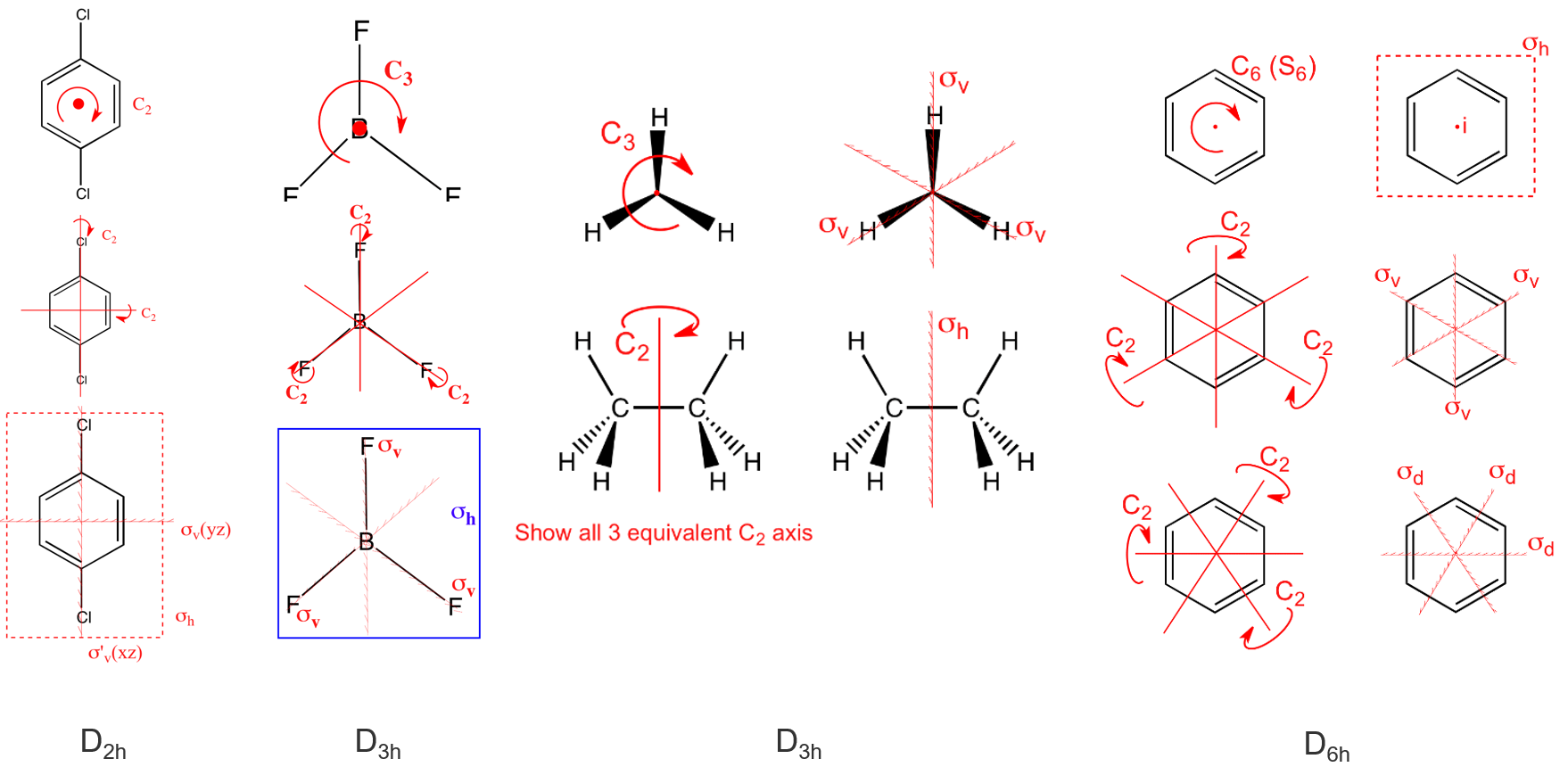(9)  $$D_{n d}$$ - contains the same symmetry elements as $$D_{n}$$ with the addition of $$n$$ dihedral mirror planes.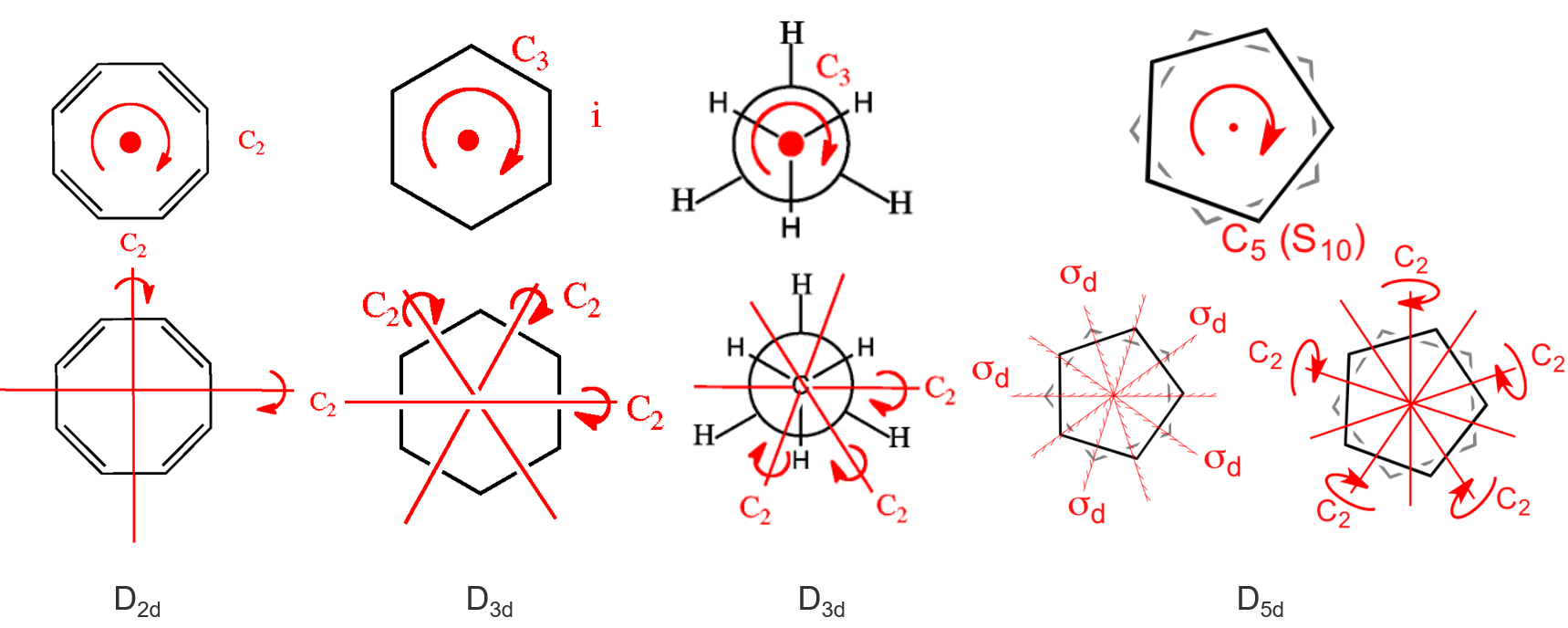(10) $$S_{n}$$ - contains the identity and one $$S_{n}$$ axis. Note that molecules only belong to $$S_{n}$$ if they have not already been classified in terms of one of the preceding point groups (e.g. $$S_{2}$$ is the same as $$C_{i}$$, and a molecule with this symmetry would already have been classified).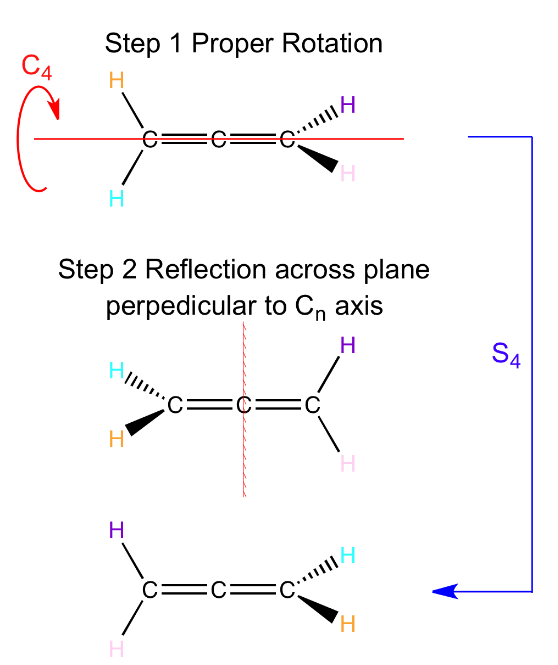The following groups are the cubic groups, which contain more than one principal axis. They separate into the tetrahedral groups ($$T_{d}$$, $$T_{h}$$ and $$T$$) and the octahedral groups ($$O$$ and $$O_h$$). The icosahedral group also exists, but is not included below. 无论是四配位四面体，还是六配位八面体，都可以

(11) $$T_d$$ - contains all the symmetry elements of a regular tetrahedron, including the identity, 4 $$C_3$$ axes, 3 $$C_2$$ axes, 6 dihedral mirror planes, and 3 $$S_4$$ axes e.g. CH4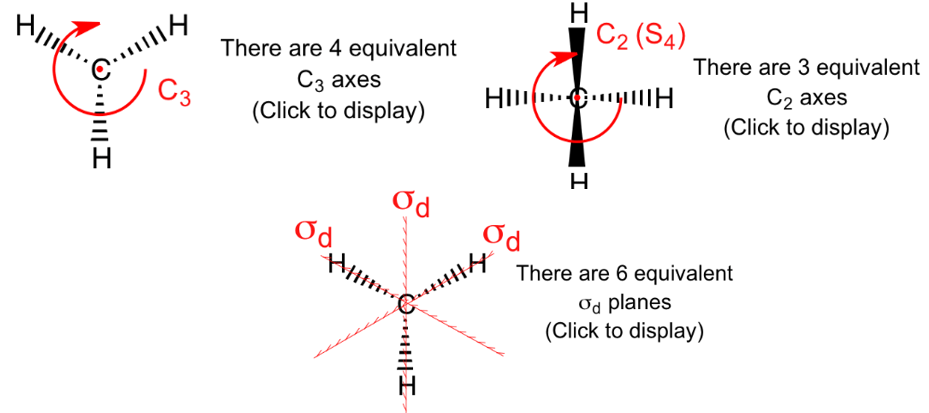(12) $$T$$ - as for $$T_{d}$$ but no planes of reflection. (very rare) [Ca(THF)6]2+

(13) $$T_h$$ - as for $$T$$ but contains a center of inversion. (very rare？)

(14) $$O_h$$ - the group of the regular octahedron e.g. SF6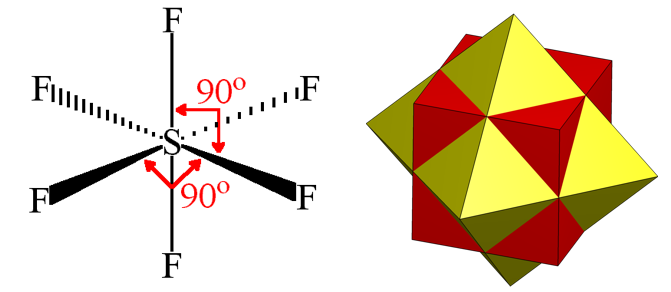(15) $$O$$ - as for $$O_h$$, but with no planes of reflection. (very rare)

The final group is the full rotation group $$R_{3}$$, which consists of an infinite number of $$C_{n}$$ axes with all possible values of $$n$$ and describes the symmetry of a sphere. Atoms (but no molecules) belong to $$R_{3}$$, and the group has important applications in atomic quantum mechanics. However, we won't be treating it any further here.

Once you become more familiar with the symmetry elements and point groups described above, you will find it quite straightforward to classify a molecule in terms of its point group. In the meantime, the flowchart shown below provides a step-by-step approach to the problem.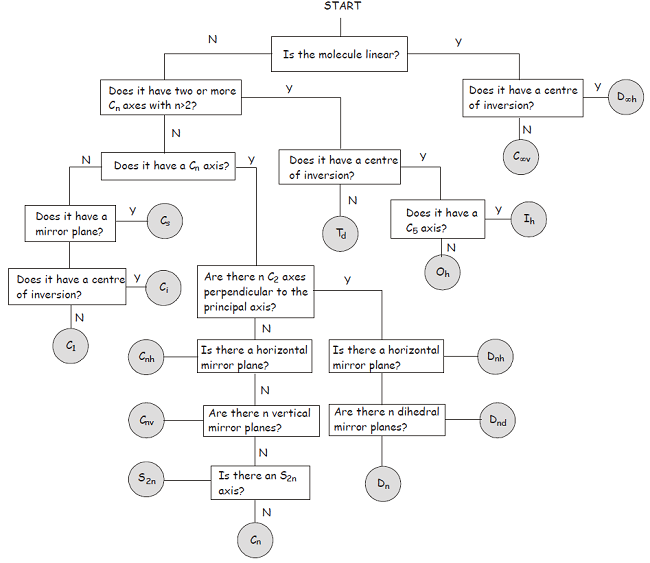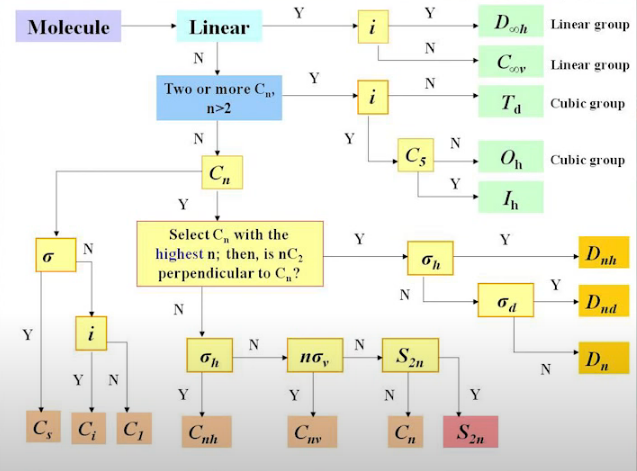Hermann-Mauguin system: Though the Hermann-Mauguin system can be used to label point groups, it is usually used in the discussion of crystal symmetry. In crystals, in addition to the symmetry elements described above, translational symmetry elements (平移对称性) are very important.
Space groups versus Point group: Translational symmetry operations leave no point unchanged, with the consequence that crystal symmetry is described in terms of space groups rather than point groups.

## Symmetry and Physical Properties

Carrying out a symmetry operation on a molecule must not change any of its physical properties.

Polarity

• For a molecule to have a permanent dipole moment, it must have an asymmetric charge distribution. The point group of the molecule not only determines whether the molecule may have a dipole moment, but also in which direction(s) it may point.
• If a molecule has a $$C_{n}$$ axis with $$n>1$$ , it cannot have a dipole moment perpendicular to the axis of rotation. Any dipole must lie parallel to a $$C_{n}$$ axis.
• The only groups compatible with a dipole moment are $$C_{n}$$  , $$C_{nv}$$  and $$C_{S}$$ . In molecules belonging to $$C_{n}$$  or $$C_{nv}$$  the dipole must lie along the axis of rotation.

Chirality

• Enantiomers (手性异构体): non-superimposable mirror images of each other, and one consequence of this symmetrical relationship is that they rotate the plane of polarized light passing through them in opposite directions.
• Chiral: cannot be superimposed on their mirror image. No symmetry elementa rotation-reflection axis $$S_{n}$$.
• 其他对称元素可能就包含了$$S_{n}$$，比如含有$$C_n$$ and $$\sigma_h$$对称元素的点群也会有$$S_{n}$$对称元素，a center of inversion is equivalent to $$S_{2}$$。
• 经验判据(As a rule of thumb)：a molecule definitely cannot have be chiral if it has a center of inversion or a mirror plane of any type ($$\sigma_h$$ , $$\sigma_v$$ or $$\sigma_d$$ ), but if these symmetry elements are absent the molecule should be checked carefully for an $$S_{n}$$ axis before it is assumed to be chiral.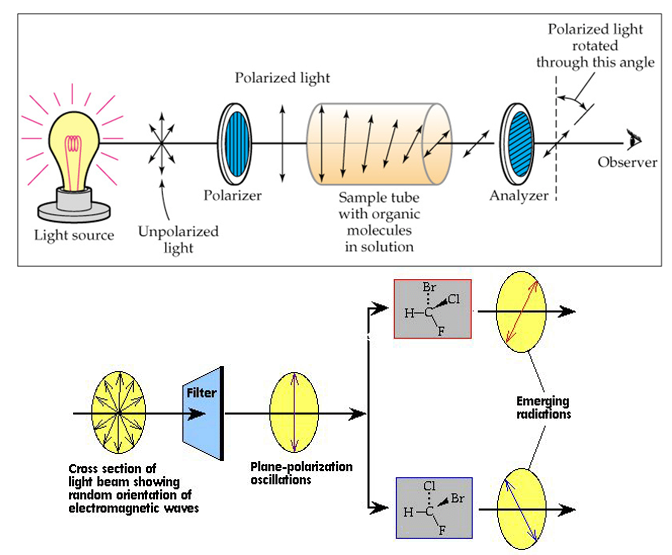(1) 手性

## Combining Symmetry Operations - ‘Group Multiplication’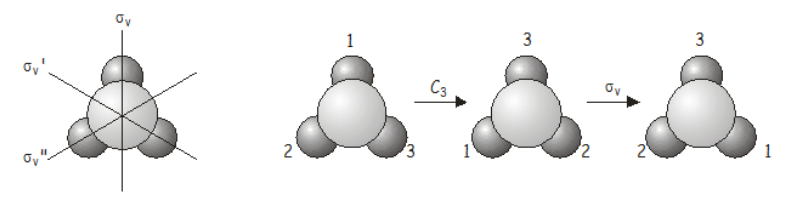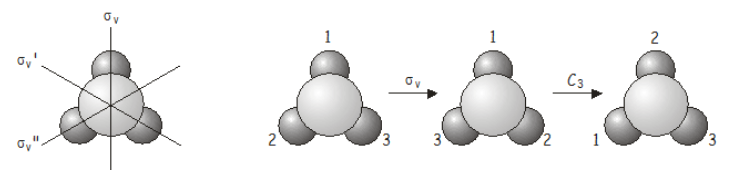There are two important points that are illustrated by this example:
(1) Order Matters，就像量子力学中的算符一样。如果对两个对称操作来说作用顺序没影响的话，那么它们就属于同一个阿贝尔群。 In some groups the symmetry elements do commute; such groups are said to be Abelian.

(2) 两次的对称操作都属于同一个point group，那么最后的结果必然等价于该point group中的另一个操作(元素)。Symmetry operations that are related to each other by other symmetry operations of the group are said to belong to the same class. 对NH3分子来说，the three mirror planes (见上图) belong to the same class (related to each other through a $$C_3$$ rotation), as do the rotations $$C_{3}^{+}$$ and $$C_{3}^{-}$$ (anticlockwise and clockwise rotations about the principal axis, related to each other by a vertical mirror plane.

The effects of applying two symmetry operations in sequence within a given point group are summarized in group multiplication tables. 下图是, the complete group multiplication table for $$C_{3v}$$. The operations written along the first row of the table are carried out first, followed by those written in the first column, 注意结果会有些许改变如果我们choose $$\sigma_{v}$$、$$\sigma_{v}^{\prime}$$和$$\sigma_{v}^{\prime \prime}$$ in some different order。## Constructing higher groups from simpler groups

A group that contains a large number of symmetry elements may often be constructed from simpler groups. This is probably best illustrated using an example. Consider the point groups $$C_2$$ and $$C_S$$. $$C_2$$ contains the elements $$E$$ and $$C_2$$, and has order 2, while $$C_S$$ contains $$E$$ and $$\sigma$$ and also has order 2 . We can use these two groups to construct the group $$C_{2v}$$ by applying the symmetry operations of $$C_2$$ and $$C_S$$ in sequence.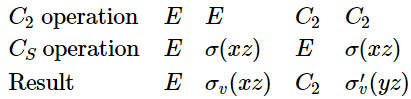Notice that $$C_{2v}$$ has order 4 , which is the product of the orders of the two lower-order groups. $$C_{2v}$$ may be described as a direct product group of $$C_2$$ and $$C_S$$. The origin of this name should become obvious when we review the properties of matrices.

## Mathematical Definition of a Group

：设G为一些元素(操作/封闭运算)gi (i = 1, 2, 3...)的集合，这个集合具有如下的性质：

• 封闭性(closure)，任何两个元素的乘积仍然属于G；
• 存在一个单位元素E，它与G中任何元素gi的乘积仍等于gi: $$\mathrm{E} \times \mathrm{g}_{\mathrm{i}}=\mathrm{g}_{\mathrm{i}} \times \mathrm{E}=\mathrm{g}_{\mathrm{i}}$$；
• 对于任何一个元素gi，必然存在一个逆元素gi-1，满足方程$$\mathrm{g}_{\mathrm{i}} \times \mathrm{g}_{\mathrm{i}}^{-1}=\mathrm{g}_{\mathrm{i}}^{-1} \times \mathrm{g}_{\mathrm{i}}=\mathrm{E}$$
比如in $$C_{3v}$$ the inverse of $$C_{3}^{+}$$ is $$C_{3}^{-}$$ , the inverse of $$\sigma_{v}$$ is $$\sigma_{v}^{\prime}$$
• 结合律(associate law)，对任何三个元素必有$$\mathrm{g}_{\mathrm{j}} \times(\mathrm{g}_{\mathrm{k}} \times \mathrm{g}_{l})=\left(\mathrm{g}_{\mathrm{j}} \times \mathrm{g}_{\mathrm{k}}\right) \times \mathrm{g}_{l}$$

The above definition does not require the elements to commute, which would require$$g_{i} g_{k}=g_{k} g_{i}$$As we discovered in the $$C_{3v}$$ example above, in many groups the outcome of consecutive application of two symmetry operations depends on the order in which the operations are applied. Groups for which the elements do not commute are called non-Abelian groups; those for which they elements do commute are Abelian.

The set of matrices representing the symmetry operations in a group obey all the conditions laid out above in the mathematical definition of a group, and using matrix representations of symmetry operations simplifies carrying out calculations in group theory.

## Review of Matrices

Matrix multiplication: Matrix multiplication is not generally commutative, a property that mirrors the behavior found earlier for symmetry operations within a point group.

Direct Products: The direct product of two matrices (given the symbol ⊗ ) is a special type of matrix product that generates a matrix of higher dimensionality if both matrices have dimension greater than one. The easiest way to demonstrate how to construct a direct product of two matrices $$A$$ and $$B$$ is by an example: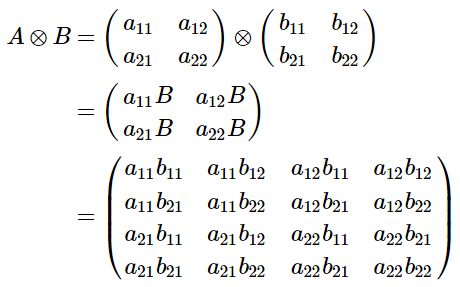Though this may seem like a somewhat strange operation to carry out, direct products crop up a great deal in group theory.

## Transformation matrices

Matrices can be used to map one set of coordinates or functions onto another set. Matrices used for this purpose are called transformation matrices. In group theory, we can use transformation matrices to carry out the various symmetry operations considered at the beginning of the course. As a simple example, we will investigate the matrices we would use to carry out some of these symmetry operations on a vector $$(x, y)$$.

The identity Operation: leaves the vector unchanged, and as you may already suspect, the appropriate matrix is the identity matrix. $$(x, y)\left(\begin{array}{ll} 1 & 0 \\ 0 & 1 \end{array}\right)=(x, y)$$

Reflection in a plane: The simplest example of a reflection matrix corresponds to reflecting the vector $$(x,y)$$ in either the x or y axes. Reflection in the $$x$$ axis maps y to $$-y$$ , while reflection in the y axis maps x to $$-x$$ . The appropriate matrix is very like the identity matrix but with a change in sign for the appropriate element.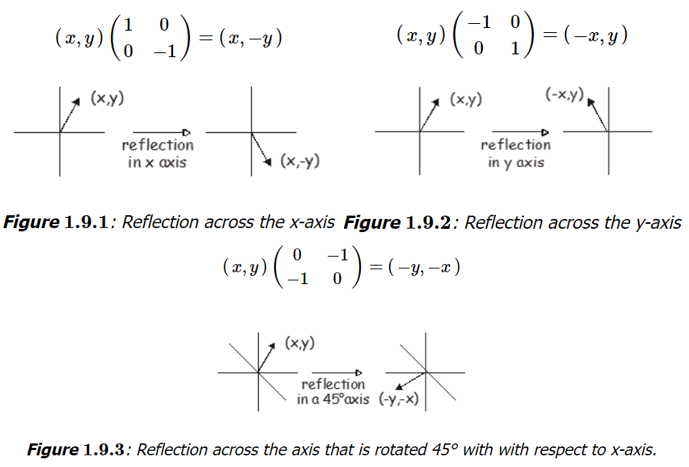Rotation about an Axis
In two dimensions, the appropriate matrix to represent rotation by an angle $$\theta$$ about the origin is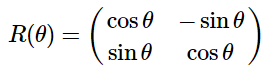In three dimensions, rotations about the $$x$$, $$y$$ and $$z$$ axes acting on a vector $$(x, y, z)$$) are represented by the following matrices.